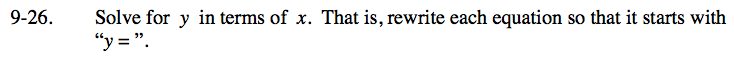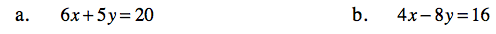### Home > CC3 > Chapter 9 > Lesson 9.1.2 > Problem9-26

9-26.
1. Solve for y in terms of x. That is, rewrite each equation so that it starts with “y = ”. Homework Help ✎

1. 6x + 5y = 20

2. 4x − 8y = 166x + 5y = 20
−6x −6x

5y = 20 − 6x

${\it y} = 4 - \frac{6}{5}{\it x}$

Use the same method as in part (a).